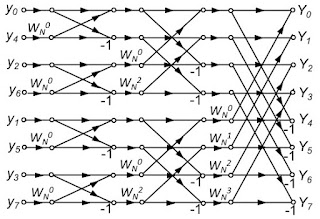## Friday, June 30, 2017

### The Fast Fourier Transform

In Chapter 11 of Intermediate Physics for Medicine and Biology, Russ Hobbie and I discuss the fast Fourier transform.
The calculation of the Fourier coefficients using our equations involves N evaluations of the sine or cosine, N multiplications, and N additions for each coefficient. There are N coefficients, so that there must be N2 evaluations of the sines and cosines, which uses a lot of computer time. Cooley and Tukey (1965) showed that it is possible to group the data in such a way that the number of multiplications is about (N/2)log2N instead of N2 and the sines and cosines need to be evaluated only once, a technique known as the fast Fourier transform (FFT).
Additional analysis of the FFT is found in the homework problems at the end of the chapter.
Problem 17. This problem provides some insight into the fast Fourier transform. Start with the expression for an N-point Fourier transform in complex notation, Yk in Eq. 11.29a. Show that Yk can be written as the sum of two N/2-point Fourier transforms: Yk = ½[Yke + Wk Yko], where W = exp(-i2π/N), superscript e stands for even values of j, and o stands for odd values.Numerical Recipes: The Art of Scientific Computing, by Press et al.
The FFT is a famous algorithm in the field of numerical methods. Below is how Press et al. describe it in one of my favorite books, Numerical Recipes.
The discrete Fourier transform can, in fact, be computed in O(Nlog2N) operations with an algorithm called the fast Fourier transform, or FFT. The difference between Nlog2N and N2 is immense. With N = 106, for example, it is the difference between, roughly, 30 seconds of CUP time and 2 weeks of CPU time on a microsecond cycle time computer. The existence of an FFT algorithm became generally known only in the mid-1960s, from the work of J. W. Cooley and J. W. Tukey. Retrospectively, we now know…that efficient methods for computing the DFT [discrete Fourier transform] had been independently discovered, and in some cases implemented, by as many as a dozen individuals, starting with Gauss in 1805!

One “rediscovery” of the FFT, that of Danielson and Lanczos in 1942, provides one of the clearest derivations of the algorithm. Danielson and Lanczos showed that a discrete Fourier transform of length N can be rewritten as the sum of two discrete Fourier transforms, each of length N/2. One of the two is formed from the even-numbered points of the original N, the other from the odd-numbered points…

The wonderful thing about the Danielson-Lanczos Lemma is that it can be used recursively. Having reduced the problem of computing Fk to that of computing Fke and Fko, we can do the same reduction of Fke to the problem of the transform of its N/4 even-numbered input data and N/4 odd-numbered data…

Although there are ways of treating other cases, by far the easiest case is the one in which the original N is an integer power of 2…With this restriction on N, it is evident that we can continue applying the Danielson-Lanczos Lemma until we have subdivided the data all the way down to transforms of length 1…The points as given are the one-point transforms. We combine adjacent pairs to get two-point transforms, then combine adjacent pairs of pairs to get 4-point transforms, and so on, until the first and second halves of the whole data set are combined into the final transform. Each combination takes on order N operations, and there are evidently log2N combinations, so the whole algorithm is of order Nlog2N.
This process, called decimation-in-time, is summarized in this lovely butterfly diagram.A butterfly diagram of a decimation-in-time Fast Fourier Transform.